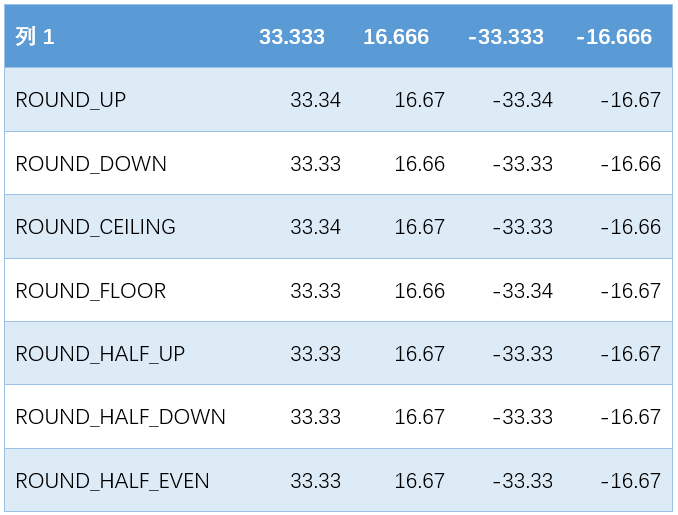• HALF_UP ：最近数字舍入(5进)。这是我们最经典的四舍五入。...小数取整 System.out.println(Math.round(1.525));//2 有向图无向图相关代码以及理解http://www.cnblogs.com/skywang12345/p/3711483.html
HALF_UP：最近数字舍入(5进)。这是我们最经典的四舍五入。HALF_DOWN：最近数字舍入(5舍)。在这里5是要舍弃的。BigDecimal b=new BigDecimal(1.125);System.out.println(b.setScale(2, RoundingMode.HALF_UP));//1.13形式取整DecimalFormat df=new DecimalFormat("#.000"); System.out.println(df.format(11.1115));//11.111小数取整System.out.println(Math.round(1.525));//2有向图无向图相关代码以及理解http://www.cnblogs.com/skywang12345/p/3711483.html
展开全文• Java 小数精确计算问题System.out.println(2.00 -1.10);//0.8999999999999999上面的计算出的结果不是0.9，而是一连串的小数。问题在于1.1 这个数字不能被精确表示为一个double，因此它被表示为最接近它的double 值，...
Java 小数精确计算问题System.out.println(2.00 -1.10);//0.8999999999999999上面的计算出的结果不是0.9，而是一连串的小数。问题在于1.1 这个数字不能被精确表示为一个double，因此它被表示为最接近它的double 值，该程序从2 中减去的就是这个值，但这个计算的结果并不是最接近0.9 的double值。一般地说，问题在于并不是所有的小数都可以用二进制浮点数精确表示。二进制浮点对于货币计算是非常不适合的，因为它不可能将1.0 表示成10 的其他任何负次幂。解决问题的第一种方式是使用货币的最小单位(分)来表示：System.out.println(200-110);//90第二种方式是使用BigDecimal，但一定要用BigDecimal(String)构造器，而千万不要用BigDecimal(double)来构造(也不能将float或double型转换成String再来使用BigDecimal(String)来构造，因为在将float或double转换成String时精度已丢失)。例如new BigDecimal(0.1)，它将返回一个BigDecimal，也即0.1000000000000000055511151231257827021181583404541015625，正确使用BigDecimal，程序就可以打印出我们所期望的结果0.9：System.out.println(new BigDecimal("2.0").subtract(new BigDecimal("1.10")));// 0.9另外，如果要比较两个浮点数的大小，要使用BigDecimal的compareTo方法。相关文档：public class Test {public static void main(String args[]) {System.out.println(Integer.toBinaryString(20));   //十进制-->2进制System ......受到“15年你积累了什么”一文的震动，特别收集该文学习Java gc关键字: java gc, 垃圾回收机制1.JVM的gc概述gc即垃圾收集机制是指jvm用于释放那些不再使用的对象所占用的内存。java语言并不要求jvm有gc，也没有规定gc如何工作。不过常用的jvm都有gc，而且大多数gc都使用类似的算法管理内存和执行收 ......JAVA文件操作总结File类File f = new File(path);path为实际路径，该路径可以是文件，或文件夹，也可以是不存在的。f.exists() 可以判断该路是否存在。f.isDirectory() 可以判断是否是文件夹。f.mkdirs(); 递归创建文件夹File和输入输出流之间纽带FileInutStream，FileOutputStreamURL url = new URL(strUr ......昨天看到这样一篇帖子，讨论类加载顺序的，也可以说是初始化顺序的，今天早上找不到了，本来想回复的，现在自己写博客回复了，呵呵public class Singleton {private static Singleton obj = new Singleton();public static int counter1 ;public static int counter2 = 0;& ......annotations : 注释好处1.文档编制2.编译器检查3.代码分析JSR 175 是编程语言的元数据工具,为将元数据合并到核心java语言中提供正式理由和说明基本知识:注释采用"@"标记形式,后面是注释名称,然后在需要数据时,通过name=value对向注释提供数据注释的三个基本种类:标记注释 ......
展开全文• Java计算数据小数截断，目前提供了下面几种方式，这些方式截断小数如下： 用的测试代码如下，里面有对几种方式的注释心得public class Test {public static void main(String[] args) {BigDecimal bigDecimal1 = new...
Java计算数据小数截断，目前提供了下面几种方式，这些方式截断小数如下：用的测试代码如下，里面有对几种方式的注释心得public class Test {public static void main(String[] args) {BigDecimal bigDecimal1 = new BigDecimal(-25);BigDecimal bigDecimal2 = new BigDecimal(7);// 无论正数或者负数都进1BigDecimal bigDecimal3 = bigDecimal1.divide(bigDecimal2, 2, BigDecimal.ROUND_UP);System.out.println("bigDecimal3:" + bigDecimal3);// 无论正数或者负数直接舍入，任何情况都不进1BigDecimal bigDecimal4 = bigDecimal1.divide(bigDecimal2, 2, BigDecimal.ROUND_DOWN);System.out.println("bigDecimal4:" + bigDecimal4);// 正数在舍入的时候都进1；负数直接舍掉BigDecimal bigDecimal5 = bigDecimal1.divide(bigDecimal2, 2, BigDecimal.ROUND_CEILING);System.out.println("bigDecimal5:" + bigDecimal5);// 正数直接舍掉，负数舍入时进1BigDecimal bigDecimal6 = bigDecimal1.divide(bigDecimal2, 2, BigDecimal.ROUND_FLOOR);System.out.println("bigDecimal6:" + bigDecimal6);// 四舍五入BigDecimal bigDecimal7 = bigDecimal1.divide(bigDecimal2, 2, BigDecimal.ROUND_HALF_UP);System.out.println("bigDecimal7:" + bigDecimal7);// 四舍五入BigDecimal bigDecimal8 = bigDecimal1.divide(bigDecimal2, 2, BigDecimal.ROUND_HALF_DOWN);System.out.println("bigDecimal8:" + bigDecimal8);// 四舍五入BigDecimal bigDecimal9 = bigDecimal1.divide(bigDecimal2, 2, BigDecimal.ROUND_HALF_EVEN);System.out.println("bigDecimal9:" + bigDecimal9);}
展开全文• ## Java小数截断

千次阅读 2017-11-21 15:37:07
本文对Java小数截断的几种方式进行了总结。

Java计算数据小数截断，目前提供了下面几种方式，这些方式截断小数如下：用的测试代码如下，里面有对几种方式的注释心得

public class Test {

public static void main(String[] args) {
BigDecimal bigDecimal1 = new BigDecimal(-25);
BigDecimal bigDecimal2 = new BigDecimal(7);

// 无论正数或者负数都进1
BigDecimal bigDecimal3 = bigDecimal1.divide(bigDecimal2, 2, BigDecimal.ROUND_UP);
System.out.println("bigDecimal3:" + bigDecimal3);

// 无论正数或者负数直接舍入，任何情况都不进1
BigDecimal bigDecimal4 = bigDecimal1.divide(bigDecimal2, 2, BigDecimal.ROUND_DOWN);
System.out.println("bigDecimal4:" + bigDecimal4);

// 正数在舍入的时候都进1；负数直接舍掉
BigDecimal bigDecimal5 = bigDecimal1.divide(bigDecimal2, 2, BigDecimal.ROUND_CEILING);
System.out.println("bigDecimal5:" + bigDecimal5);

// 正数直接舍掉，负数舍入时进1
BigDecimal bigDecimal6 = bigDecimal1.divide(bigDecimal2, 2, BigDecimal.ROUND_FLOOR);
System.out.println("bigDecimal6:" + bigDecimal6);

// 四舍五入
BigDecimal bigDecimal7 = bigDecimal1.divide(bigDecimal2, 2, BigDecimal.ROUND_HALF_UP);
System.out.println("bigDecimal7:" + bigDecimal7);

// 四舍五入
BigDecimal bigDecimal8 = bigDecimal1.divide(bigDecimal2, 2, BigDecimal.ROUND_HALF_DOWN);
System.out.println("bigDecimal8:" + bigDecimal8);

// 四舍五入
BigDecimal bigDecimal9 = bigDecimal1.divide(bigDecimal2, 2, BigDecimal.ROUND_HALF_EVEN);
System.out.println("bigDecimal9:" + bigDecimal9);

}

展开全文Round
• 小数精确计算System.out.println(2.00 -1.10);//0.8999999999999999上面的计算出的结果不是 0.9，而是一连串的小数。问题在于1.1这个数字不能被精确表示为一个double，因此它被表示为最接近它的double值，该程序从2...
• 2016-09-01 01:19最佳答案楼上的全不明白楼主的意思，楼主要的...严重BS楼上的，尤其是说java语言的那位。我来告诉你这个问题用递推解决首先要你承认一个公式，我是习惯pascal语言的，c++怕写错，反正只是算法，你忍...
• 本文实例为大家分享了java指定精确小数位的具体代码，供大家参考，具体内容如下java代码:public class App2 {public static void main(String[] args) {String val = checkNumber("10.1234155", 2, 6).toString();...
• ## java小数计算

千次阅读 2019-02-24 21:19:51
我也知道小数使用double与float会造成精度问题，但是一直都没有关心到底应该如何解决，最近工作中碰到了一个上千万的小数计算，才发现使用double计算小数不仅仅精度会错，在数值过大的情况下会变成科学计数法表示。...
• 小数保留后k位方法 import java.util.Scanner;//输入 import java.math.BigInteger;//大整数类 import java.math.BigDecimal;//大浮点数类 public class Main { public static void main(String[] args) { ...
• 例：new java.text.DecimalFormat(”#.00″).format(3.1415926) #.00 表示两位小数 #.0000四位小数 以此类推… 方式三： double d = 3.1415926; String result = String .format(”%.2f”); %.2f %. 表示 小数点前...
• java 两个整数相除保留两位小数java中，当两个整数相除时，由于小数点以后的数字会被截断，运算结果将为整数，此时若希望得到运算结果为浮点数，必须将两整数其一或是两者都强制转换为浮点数。 例如： (float)a/b...
• 同样，大多数开发人员也容易忽略java.math.BigDecimal 所提供的任意精度的小数 ―大多数应用程序不使用它们。然而，在以整数为主的程序中有时确实会出人意料地需要表示非整型数据。例如，JDBC使用 B...
• import java.math.BigDecimal; /** * 类描述： * @version 1.0 * @author chenairu * */ public class TM { public static void main(String[] args) { ...
• 感谢大家积极回答！目前的解决办法数据库类型设置timestamp类型的length=0页面输入...小数秒(毫微秒)哈哈我说的输入其实就是一个日期控件选择日期，因为需要给用户修改日期。下面是sql:CREATETABLE"public"."tb_aaa...
• Java中的小数运算与精度丢掉float、double类型的问题咱们都知道，核算机是运用二进制存储数据的。而往常日子中，大多数情况下咱们都是运用的十进制，因而核算机显示给咱们看的内容大多数也是十进制的，这就使得许多...
• 保留两位小数{ 方法一：{  double c=3.154215;  Java.text.DecimalFormat myformat=new java.text.DecimalFormat("0.00");  String str = myformat.format(c);  } 方式二：{  java.text....
• float、double类型的问题我们都知道，计算机是使用二进制存储数据的。而平常生活中，大多数情况下我们...我们先来看看十进制小数转二进制小数的方法对小数点以后的数乘以2，会得到一个结果，取结果的整数部分(不是1...
• 从标准输入中输入一个小数，编写程序将其转换成相应的分数显示，即转换为几又几分之几。 要求： 1、输入的小数包括整数部分、小数点和小数部分；整数部分和小数部分最多有7位数字；整数部分可以为0，若整数部分大于...
• 对于正小数而言，可以考带+号，并考虑两种情况，第一个数字为0和第一个数字不为0，第一个数字为0时，则小数点后面应该不为0，第一个数字不为0时，小数点后可以为任意数字5. 对于负小数而言，必须带负号，其余都同.....
• 求整数除法小数点后第n位开始的3位数位数不足的补0,如0.125小数第3位后三位:0.12500→500输入格式:a b n,空格分开,a是被除数,b是除数,n是小数后的位置输出格式:3位数字,a÷b小数后第n位开始的3位数字样例:输入:1 8 1...
• public classTest {//在java中如何把小数转化成百分数public static void main(String[] args) {NumberFormat num = NumberFormat.getPercentInstance();num.setMaximumIntegerDigits(3); //小数点前面...
• import java.util.Scanner;public class ChangeToFenshuDemo {public static int getGongYueShu(int a, int b) {//求两个数的最大公约数int t = 0;if(a < b){t = a;a = b;b = t;}int c = a % b;if(c == 0){return...
• 小编典典如果使用double或float，则应使用舍入或期望看到一些舍入错误。如果你无法执行此操作，请使用BigDecimal。你遇到的问题是0.1不是精确的表示形式，并且通过执行两次计算，你正在使该错误复杂化。...
• import java.math.BigDecimal;import java.math.RoundingMode;import java.text.DecimalFormat;import java.text.NumberFormat;public class DoubleTest {/*** 保留两位小数，四舍五入的一个老土的方法* @pa...
• Java在做变量自等的时候，可能会发生保留小数位数不足的情况。如下代码，保留3位小数，实际运行中，发现有些结果是2位的，有些是3位的。avg = sum_time/count; avg = (double)(Math.round(avg * 10000)/10000.0); ...
• 导读热词下面是编程之家 jb51.cc 通过网络收集整理的代码...import java.text.DecimalFormat;public class NumberUtil {public void roundNumber(double numberToRound) {DecimalFormat df = new DecimalFormat("0....
• 例子： 0.813*2 整数部分: 1 小数部分: 0.626 0.626*2 整数部分: 1 小数部分: 0.252 0.252*2 整数部分: 0 小数部分: 0.504 0.504*2 整数部分: 1 小数部分: 0.008 0.008*2 整数部分: 0 小数部分: 0.016 代码实现： ...
• ## java小数精度问题

千次阅读 2018-05-31 16:36:22
1、原因由于浮点数在转化为二进制时无法运算完结，所以会无限循环下去，导致运算结果达不到期望值。例如：1.14，转化为二进制，然后再转回十进制就变成了：1....import java.math.BigDeci...
• double num1 = 1.0; double num2 = 0.8; BigDecimal a = new BigDecimal(String.valueOf(num1)); BigDecimal b = new BigDecimal(String.valueOf(num2)); BigDecimal bigDecimal = a.subtract(b);......

# java小数java 订阅